## Spring Design

Design information for engineers, such as spring calculation formulas,

which are the basis of spring design, can be found here.

### Meaning of Symbols

The symbols used for spring design are shown in Table 1 below. The values of the longitudinal elastic modulus (E) are based on Table 2.

Table 1. Symbols and units used in calculations

Symbol Meaning Unit
d Material diameter mm
Di Coil inner diameter mm
D0 Coil outer diameter mm
D Coil average diameter　D=( Di + D0)/2 mm
ΔD Reduction in coil average diameter with load applied mm
N Number of coils
v Poisson’s ratio of materials（0.3)
c Spring index　c=D/d
E Longitudinal elastic modulus GpaまたはN/mm2
l Second moment of area
Z Section modulus
P(P1, P2) Load (Force) applied to the spring N
M Torsion moment (Torque) acting on the spring N・mm
a1, a2 Arm length mm
L Active extension length of the spring mm
kr (krd) Spring constant N・mm/rad(N・mm/deg)
φ(φd) Spring torsion angle rad(deg,°)
R(R1, R2) Load radius mm
Ds Guide stud diameter mm
kb Bending stress correction factor
σ Bending stress Gpa or N/mm2

Table 2.Longitudinal elastic modulus：E（N/m㎡）

Material E Value
Spring steel material
Hard steel wire
Piano wire
Oil-tempered wire
206×103
Stainless steel SUS302

SUS304

SUS631

186×103
186×103
196×103
Brass Wire 98×103
Nikel-silver wire 108×103
Phosphor bronze wire 98×103
Beryllium copper wire 127×103

### Basic Calculation Formula Used for Design### Design of Torsion Springs

The torsion coil spring must be designed in consideration of the bending deflection that occurs in the arm which extends from the coil part.

The necessity to consider the arm part can be judged with the following formula.
If a1 + a2 is 0.09π DN or more, it is recommended to consider the arm length.Figure 2. Arm Length of Torsion Springs

The spring constant (KT) of the torsion springs can be calculated with the following formula.

When the Length of the Arm Is NegligibleWhen the Length of the Arm Is Not NegligibleIn addition, any stress (σ) can be calculated by the following formula.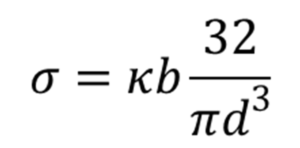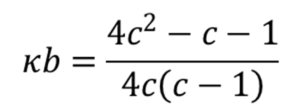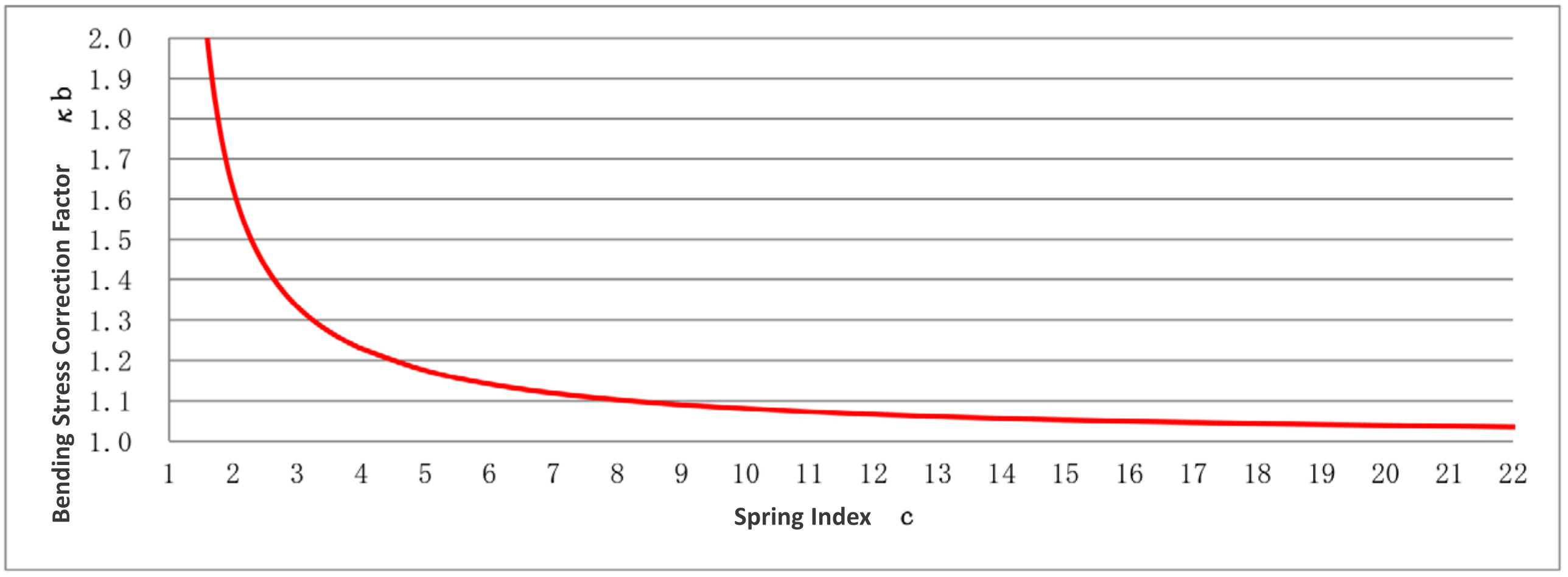Figure 3. Relationship between Spring Index “c” and Bending Stress Correction Factor “κb”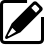Product information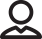Our Proposals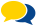Popular questions